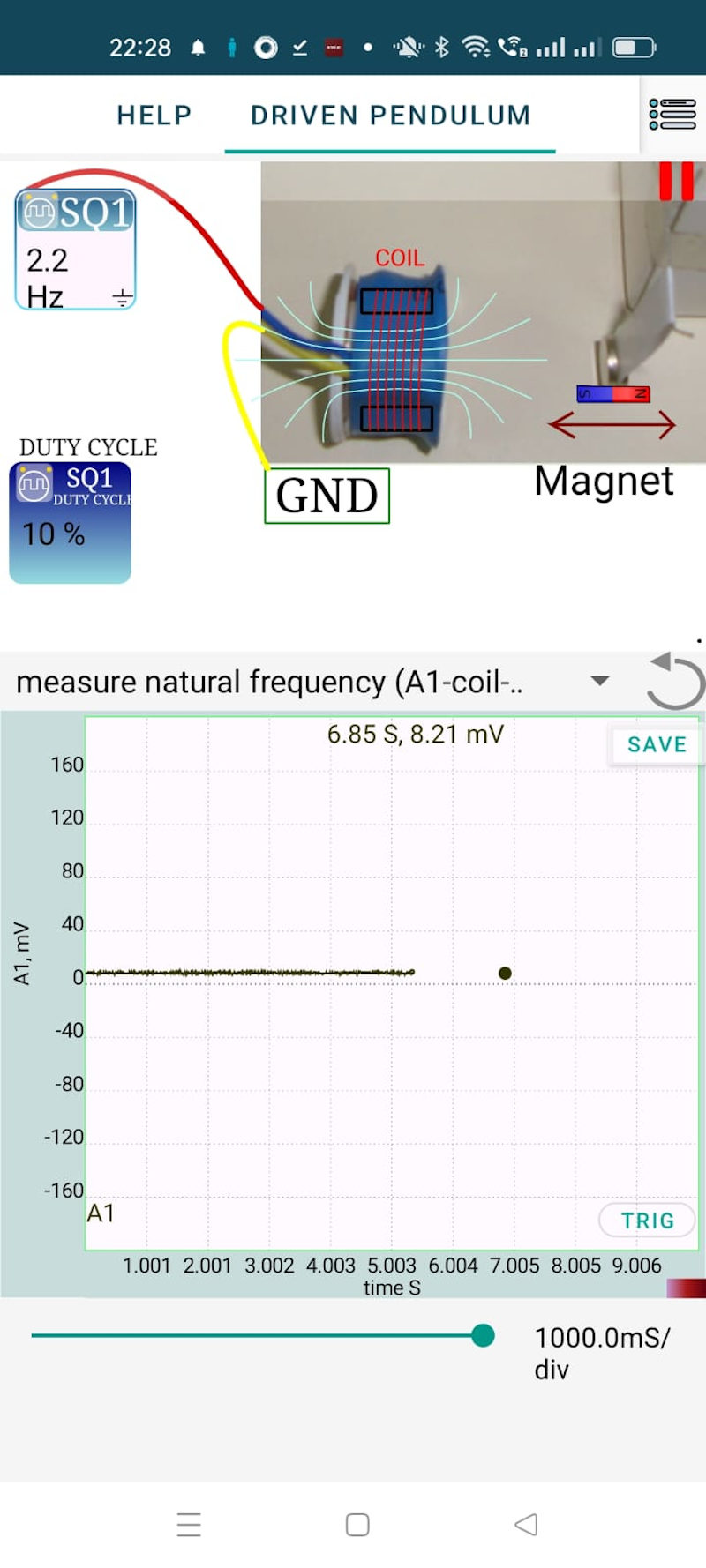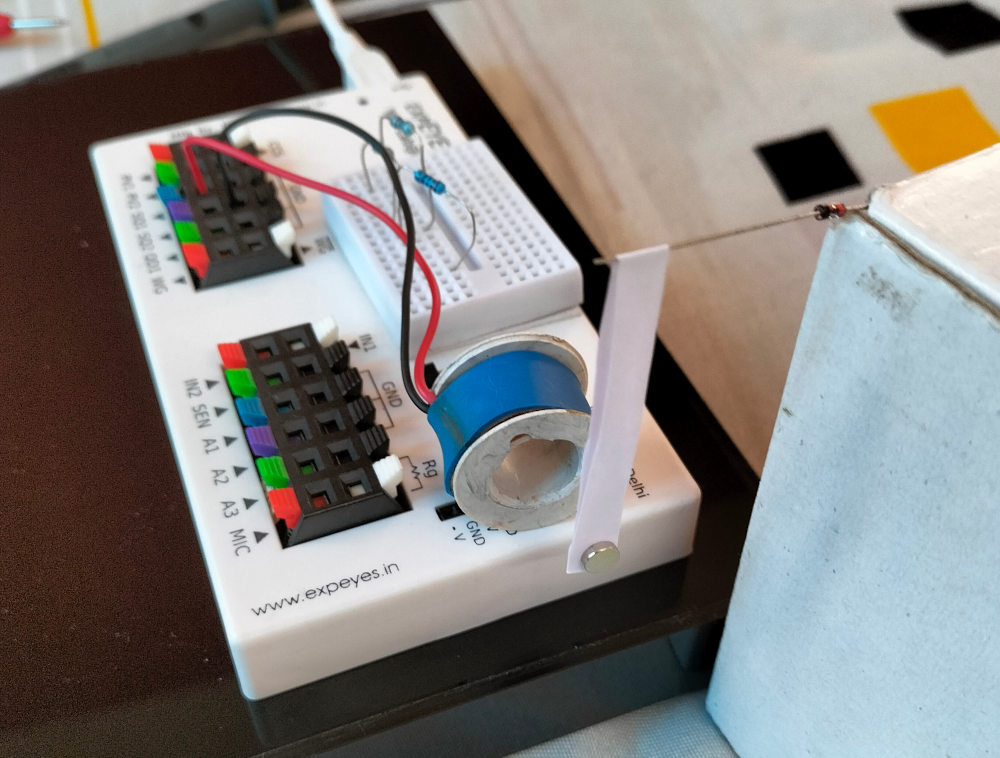# ExpEYES

## Driven Pendulum, Resonance

### Oscillations

Oscillating systems transfer energy between two different storage modes, kinetic and potential in thecase of a pendulum. For LC circuit oscillators the energy is tranferred between the Capacitor and the Inductor. Every oscillating system has a natural resonant frequency, and it depends on the oscillating elements and the forces involved. For a pendulum, it is the length and the value of acceleration due to gravity.

### Driven Oscillations

Once started, the oscillations grdually die out due to the damping forces, unless some energy is supplied to it continuously. If the supplied energy is periodic and it’s frequency and phase matches with the natural resonant frequency of the system, maximum energy transfer will occur and the amplitude of oscillations will drastically increase. This phenomena is called resonance.

• Construct a pendulum using two button shaped magnets and a strip of paper, as shown in the figure.
• Calculate the period using $T=2\pi\sqrt{\frac{L}{g}}$. For a 5cm long pendulum, the resonant frequency ($\frac{1}{T}$) is around 2.2 Hz.
• Connect the coil from SQ1 to Ground.
• Place the pendulum near the coil, as shown in the figure.
• Adjust the frequency of SQ1 to maximize the amplitude of oscillations.

Watch a Video of Driven Pendulum at Resonance